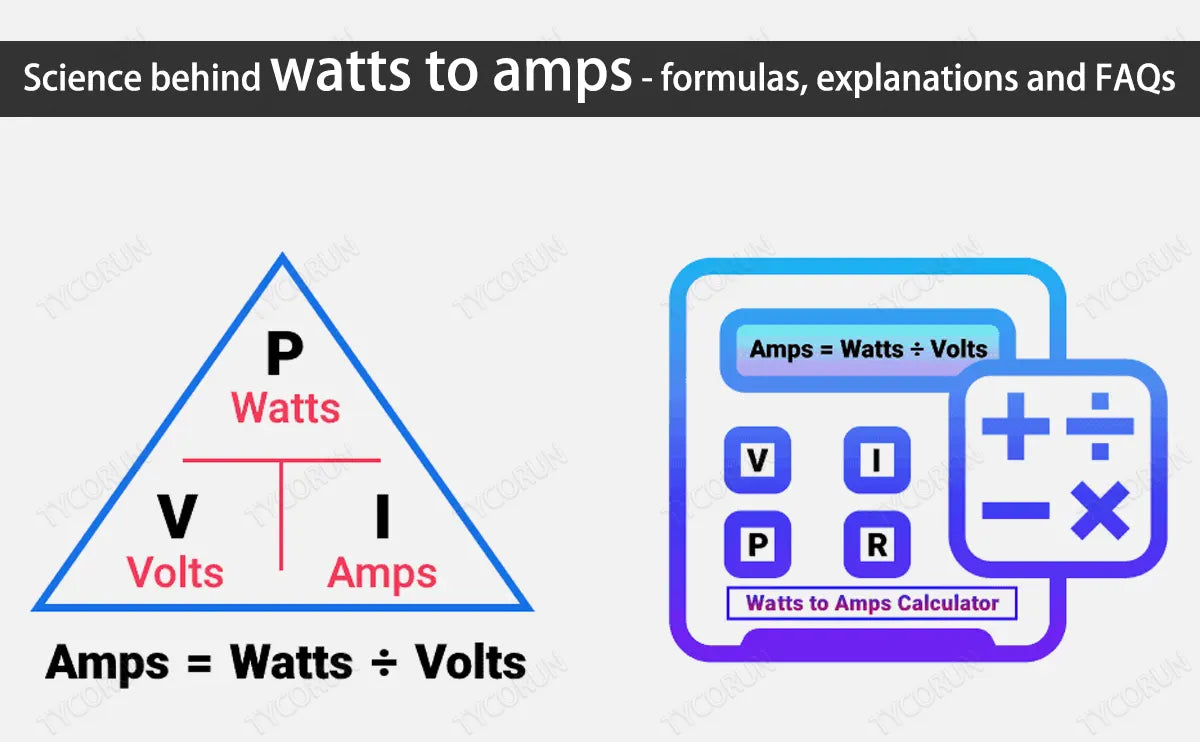Electricity powers our modern world, lithium battery 12v 100ah  is everywhere in our lives，but understanding the terminology that accompanies it can be a bit like deciphering a foreign language for many people. Terms like watts, amps, volts, AC, DC, ohms, and amp-hours are thrown around, often leaving us bewildered. In this article, we'll shed light on these electrical concepts, explore watts to amps and how they relate to each other, and provide practical tips for conversions and calculations.

## 1. What are watts, amps, volts, AC and DC?

Watts:

In the International System of Units (SI), the watt is the unit of power or electric flux. Watt is represented by W. James Watt (1736 – 1819) a Scottish engineer who invented this phenomenon while working on his steam engine. His invention was fundamental for many researches on electricity.

• An amount of power an electrical device is using.
• The rate at which electromagnetic energy is dissipated or absorbed is called watt.
• In DC and AC systems, power is a product of current and voltage.
W = current (I) x voltage (V)

• Power (watt) is also proportional to the ratio of the square of the voltage to the resistance.

(Watt) P = V2/R

• Power is also proportional to the product of the square of the current and resistance.

(Watt) P = I2 R

Amps:

What are amps? The word Ampere is after the name of a French Physicist and mathematician Andre Marie Ampere in 1775- 1836. The SI Unit of the ampere is Coulomb represented by C.

• 1: 1 ampere of current is stated that one coulomb of charge i.e. 6.24x 1018 carried through a certain point in one second.
• 2: An ampere is the rate of current passing through a circuit measured in amperes represented with A.

1A =1 C/s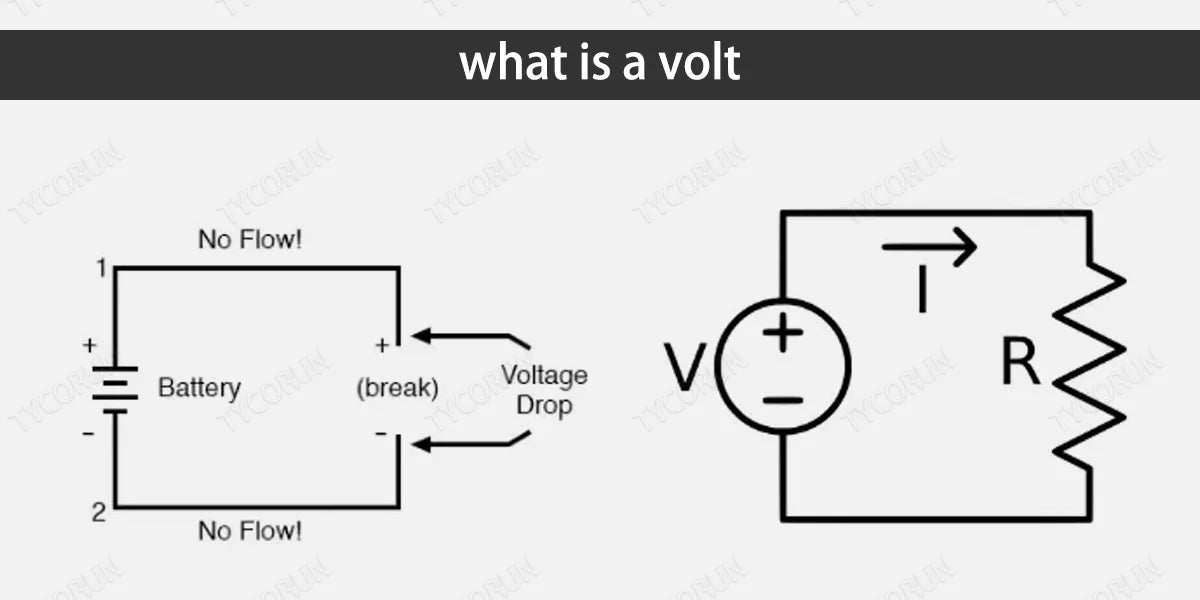Volts:

In simple words, it is the pressure or speed of current flow that is created by the potential difference between two opposite points of the circuit. The best example of this potential difference is a water tank. The higher and wider tank causes the water to flow outside with greater pressure.

Voltage:

Voltage is the pressure that pushes the current through a circuit that enables a device to do work.
The word voltage is after the name of Italian Alessandro Volta (1745- 1827) who invented a device to measure the volts which help us today in our household battery system. It is represented by V. Voltage can be either AC or DC.

Voltage in Ac:
In AC it flows in a wavy form and reverses its direction at regular intervals.

Voltage in DC:
While in DC it flows in one direction steadily.

AC (Alternating Current):
It is a type of current in which the direction of electrons changes back and forth at regular intervals i.e. this current starts from zero reaches its climax and then falls to zero, and reverses its direction in the same way. It is abbreviated as AC. Usually, this current flows in sine waves in most of the circuits.

This current is supplied from the grid lines and generators to the business places and houses. Ac had the advantage over DC for decades due to its ability to transfer current for long distances with less resistance and loss of energy.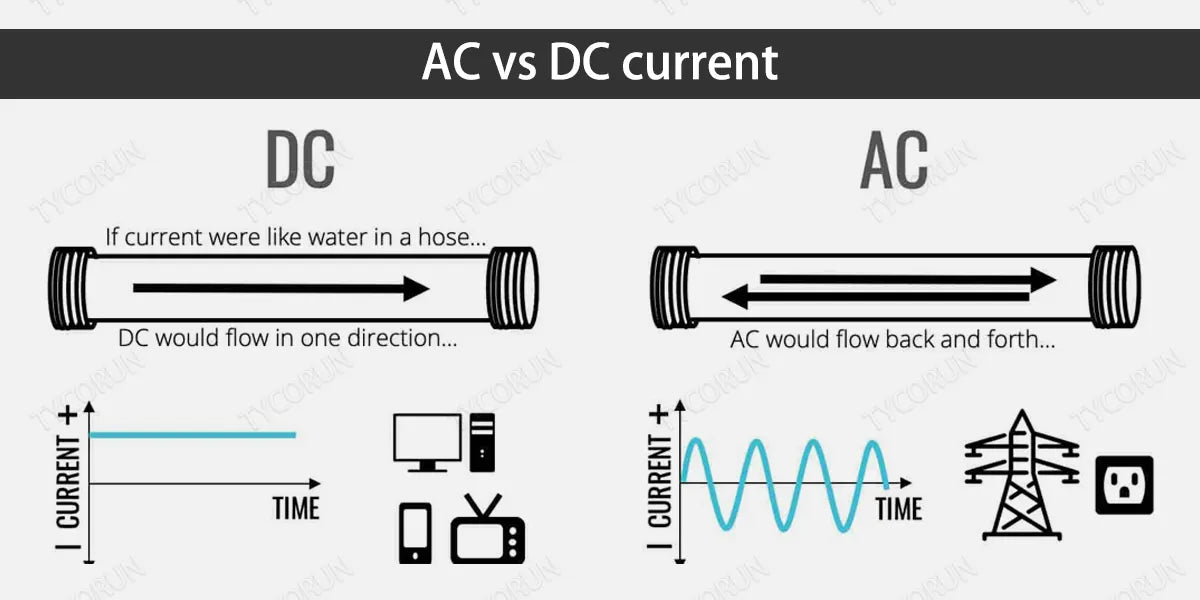DC (Direct Current):
In the early days of the invention of the current, DC was called galvanic current.
It is a type of current in which electric charge flows in one direction. The constant flow of current distinguishes DC from AC. This current may pass through a conductor, semiconductor, insulator, and even vacuum.

A rectifier is used to convert AC into DC which allows current to flow in one direction. Likewise, DC is converted to AC by using an 2000w pure sine wave inverter.

It is used in battery systems to be supplied for large power electric systems. For the installation of a DC system in homes or offices, different types of switches, connectors, and sockets are used to maintain the lower voltage and higher current for the same power as that is produced by the AC system.

## 2. How to calculate amps, volts, and watts? How many watt are in an amp?

• How many watts are in an amp?
Amps = Watts/ Volts
Volts = amps x ohm
Watts = Amps x Volts
• How many Watts are in an ampere?
Watts = amp x Volts
eg.1A x 120v = 120 W

## 3. Differences between amps, volts,watts, ohm, amp hour and their relate to each other?

Firstly, we will see mathematical equations of all these phenomena in the field of electricity to understand the relationship between them.

Ampere: I = V/R (Voltage by resistance)
Volts: V= I x R (Voltage times current)
Watts: W=I x V (current multiplied by volts)

To study all these mathematical equations of electricity Ohm’s Law provides a base to understand these three equations. Watts, amps, and voltage culminate into Ohm’s law. It describes the relationship between voltage, current, and resistance. If you want to know the your car battery voltage, you can also use this formula to calculate. Ohm uses the formula of resistance

Ohm: R= V/I (voltage by current)

it states that:

• 1: Current is directly proportional to the potential difference between two points.
• 2: Resistance of different metals is different when current passes through them. Although all factors (watts, volts, and amps) have different functions but are strongly interrelated with each other.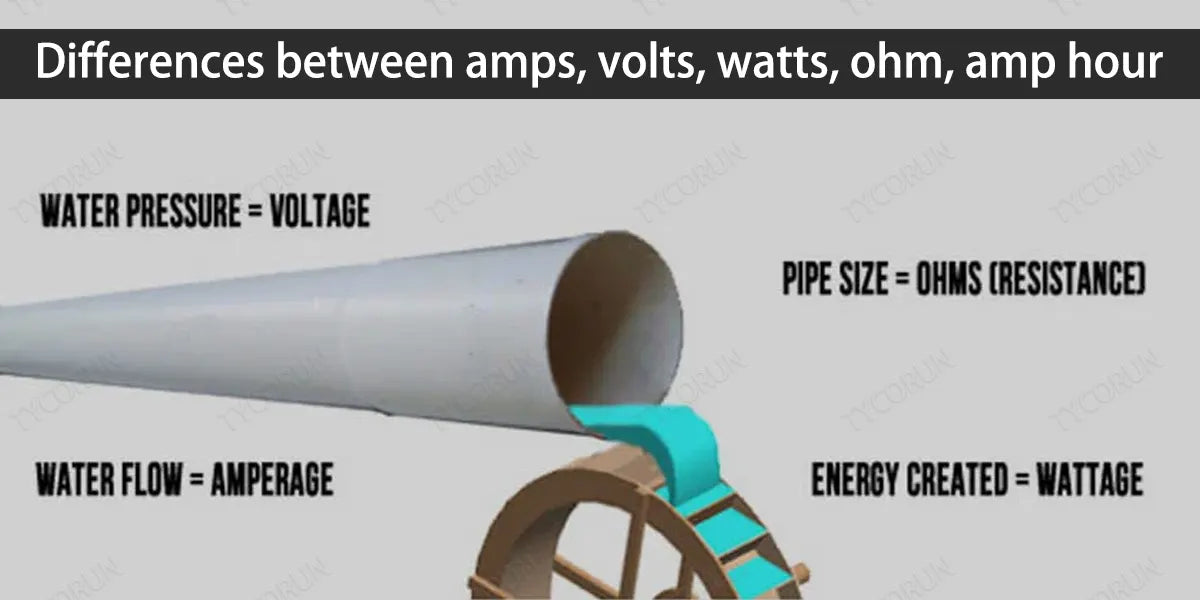## 4. What does ampere hour mean in batteries

Ampere Hour:

Ampere hour is abbreviated as Ah. The amount of 1 ampere of current flows for 1 hour through a battery is called ampere-hour or Ah.

In another way, it is stated that the amount of amperage a battery can provide for one hour. This unit is useful for calculating the storage of the batteries. Large batteries are rated in Ah. In small batteries, it can be calculated as mAh.

Determining a battery's amp hour rating

One ampere of current represents 1 coulomb of electrical charge moving past in one second. In an hour, the amount of charge passed through a battery is 3,600 coulombs.

Mathematical representation of Ampere hour:

Amp hour (Ah) = Current (I) x Discharge time (T)

## 5. How to convert watts to amps? Can I convert amps to watts manually?

To convert the watts into amps, there is a formula to apply.

Ampere= watts/ volts

we can also convert amps to watts by using the related formula

i.e. Watts = Amps x volts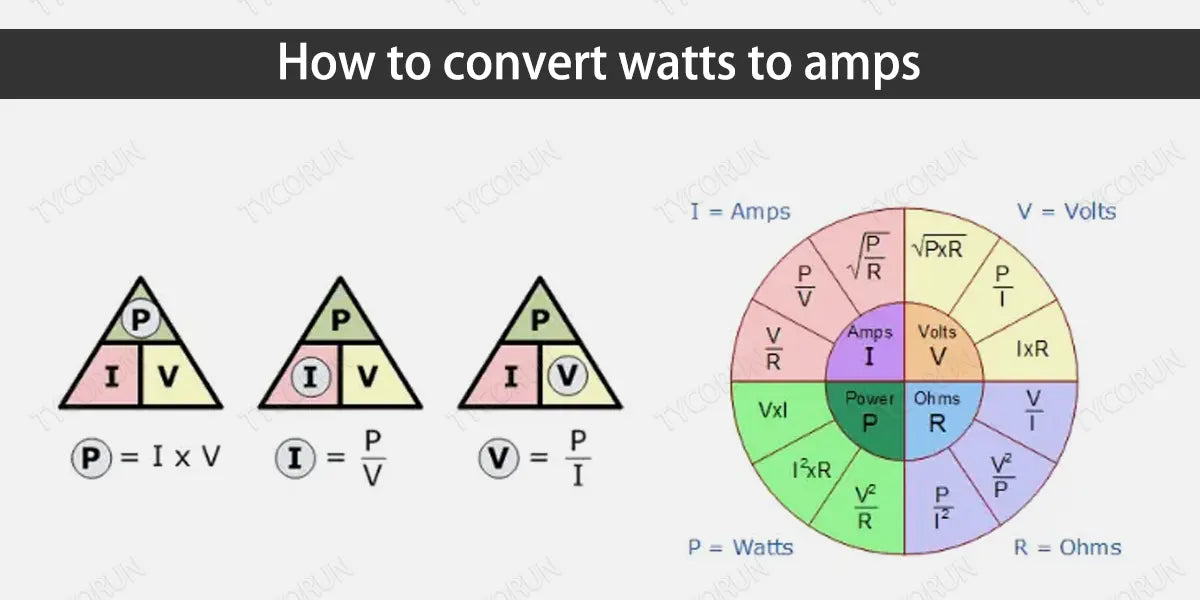### ① DC circuits

When the current is Dc there is no power factor involved;

For example: Amps = Watts/ volts = 100/120 = 0.83 A

### ② AC circuits

When the current is alternating then the power factor involves in the system.

Understanding of Power Factor:

The power factor of an AC system is defined as the ratio of the real power absorbed by the conductor to the apparent power passing in the circuit. The maximum power factor is 1.00, which means that 100% of the power delivered to the machine is active power converted into useful energy.

For example: Amps = Watts /volts x p.f =100 / 120 x 1= 0.83 A

## 6. Watts to amps chart

### ① 120V

 Watts Amperes 10 watts 0.083 A 20 watts 0.167 A 30 watts 0.250 A 40 watts 0.333 A 50 watts 0.417 A 60 watts 0.500 A 70 watts 0.583 A 80 watts 0.667 A 90 watts 0.750 A 100 watts 0.833 A 200 watts 1.667 A 300 watts 2.500 A 400 watts 3.333 A 500 watts 4.167 A 600 watts 5.000 A 700 watts 5.833 A 800 watts 6.666 A 900 watts 7.500 A 1000 watts 8.333 A

### ② 240V

 Watts Amps 100 watts 0.42 A 200 watts 0.83 A 300 watts 1.25 A 400 watts 1.67 A 500 watts 2.08 A 600 watts 2.5 A 700 watts 2.92 A 800 watts 3.33 A 900 watts 3.75 A 1000 watts 4.17A 1100 watts 4.58 A 1200 watts 5 A 1300 watts 5.42 A 1400 watts 5.83 A 1500 watts 6.25 A 1600 watts 6.67 A 1700 watts 7.08 A 1800 watts 7.5 A 1900 watts 7.92 A 2000 watts 8.33 A 2500 watts 10.42 A 3000 watts 12.5 A

## 7. How to convert amps to watts?

### ① DC circuits

In the Dc circuit, there is no power factor involved

For example: Watts = amps x Volts = 3 x 240 = 720 W

### ② AC circuits

In an Ac circuit, there is a power factor involved which varies from 0 to 1.

For example: Watts = amps x volts x p.f =3 x 240 x 1 = 720 W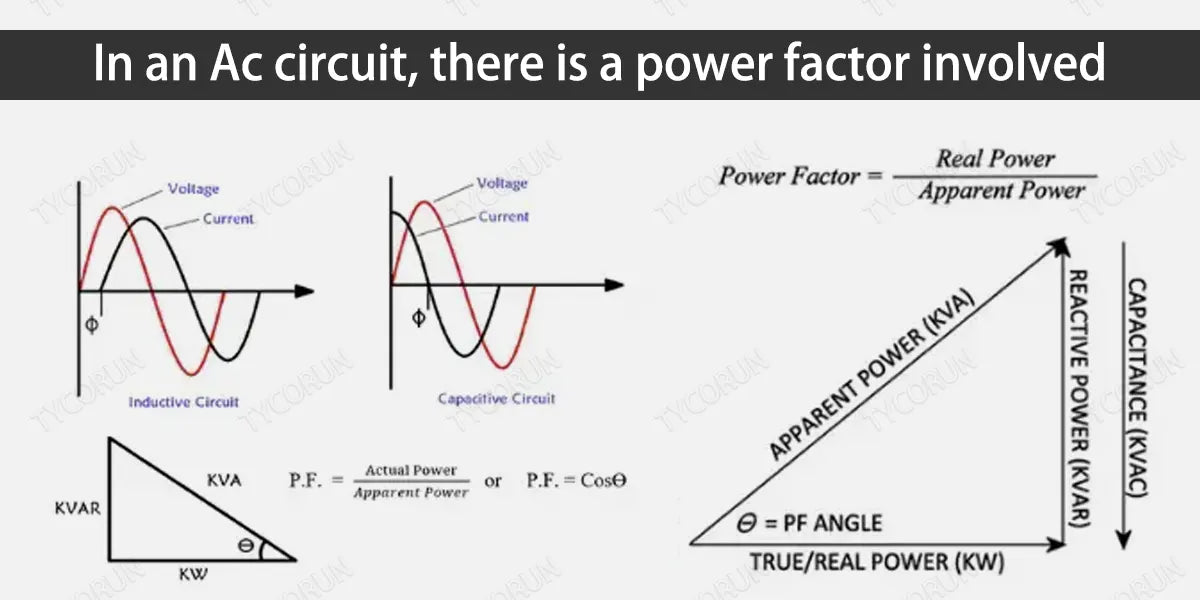## 8. What is the function of watts and amps?

Watt: In the field of electricity, and home appliances watts matter a lot for days and nights. Watts are significant to all electric appliances and a watt is a unit of power. Or watt is the rate at which energy is generated or dissipated. And the Amp is used to measure the electric current. Electrical appliances usually have a maximum charging current that cannot be exceeded.

## 9. FAQs about watts to amps

### ① How many amps is 60 watts?

If the voltage is 120v:

Amps = Watts/volts = 60 / 120 = 0.5 A

### ② How many watts is a 2.4 amp charger?

If the voltage is 5v:

Watts = amps x volts = 2.4 x 5 =12 W

### ③ What is 1500 watts to amps?

If the voltage is 124v:

Amps= Watts/Voltage =1500/124= 12.5 A

### ④ Does high amps mean high watts?

Yes, under the premise of constant voltage, high amps mean high watts (Power) because A and W are directly proportional to each other.

Related articles: battery capacity